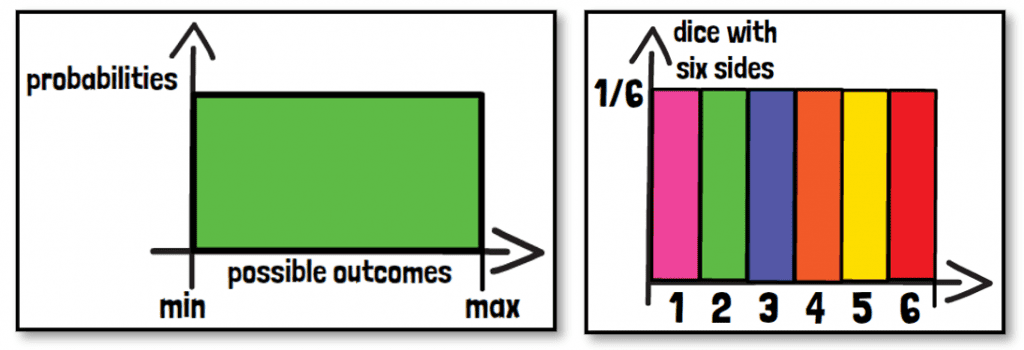# What is the uniform distribution? (approx. 5 min.)The uniform distribution is probably the simplest of all probability distributions. It is used for data, where all outcomes within a predefined interval have the same probability of occurring. The only parameters of this distribution are the minimum and maximum value of this interval.

Some uniform distributions are continuous. Even though there is an infinite number of outcomes within the range of these, they all have an equal probability of occurring. An example for a discrete uniform distribution would be the following. A dice with six sides is rolled 100 times. The probability of all six sides, assuming this is an unbiased dice, would be the same. The outcome possibilities would be 1 to 6, the probability is 1/6th for all outcome possibilities equally. For a dice with 10 sides, the outcomes are all possibilities from 1 to 10. The probability is lower for each outcome, since the overall probability of 1 is divided by the 10 outcome possibilities, instead of six like before.

The uniform distribution can be plotted as one straight horizontal line if the data is continuous. In case of discrete data, it can be plotted as a bar graph, where it consists of bars that all show the same height for all potential outcomes. Therefore, it is also called the rectangular distribution. Since the overall probability sums to 1, the height of the bars decreases with increasing interval range. The height of the bars is always 1 divided by the number of possible outcomes. The real distribution does not show perfect equally high bars as shown before, they range around the expected probability.

If you are interested in a visual presentation of this topic, please feel free to follow us on our GCP Mindset YouTube channel! If you would like to know more about how we could implement statistics in your clinical trial, send us a mail to statistics@gcp-service.com.